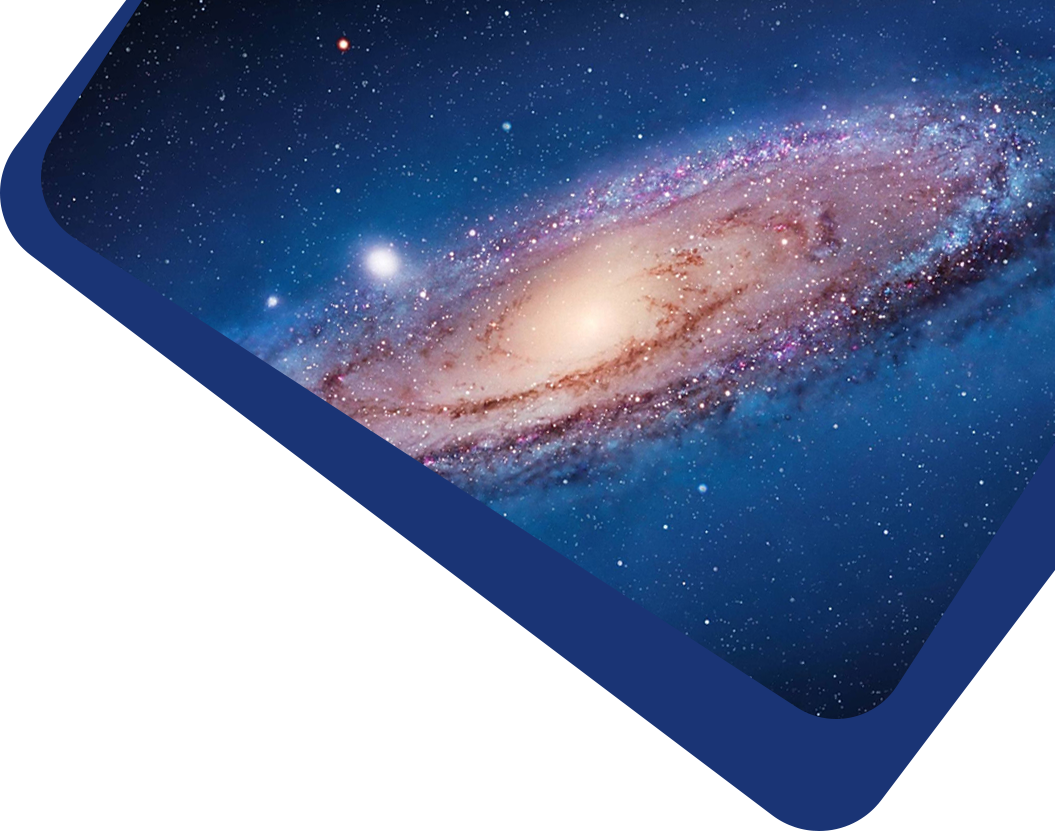Vol 21, No 12

## Characterizing microlensing planetary system OGLE-2014-BLG-0676Lb with adaptive optics imaging

#### Xiao-Jia Xie, Subo Dong, Yossi Shvartzvald, Andrew Gould, Andrzej Udalski, Jean-Philippe Beaulieu, Charles Beichman, Laird Miller Close, Calen B. Henderson, Jared R. Males, Jean-Baptiste Marquette, Katie M. Morzinski, Christopher R. Gelino

Abstract

Abstract We constrain the host-star flux of the microlensing planet OGLE-2014-BLG-0676Lb using adaptive optics (AO) images taken by the Magellan and Keck telescopes. We measure the flux of the light blended with the microlensed source to be K = 16.79 ± 0.04 mag and J = 17.76 ± 0.03 mag. Assuming that the blend is the lens star, we find that the host is a $$0.73^{+0.14}_{−0.29} M_{\odot}$$ star at a distance of $$2.67^{+0.77}_{−1.41}$$ kpc, where the relatively large uncertainty in angular Einstein radius measurement is the major source of uncertainty. With mass of $$M_p = 3.68^{+0.69}_{−1.44} M_J$$ , the planet is likely a “super Jupiter” at a projected separation of $$r_{\perp} = 4.53^{+1.49}_{−2.50}$$ AU, and a degenerate model yields a similar $$M_p = 3.73^{+0.73}_{−1.47} M_J$$ at a closer separation of $$r_{\perp} = 2.56^{+0.84}_{−1.41}$$ AU. Our estimates are consistent with the previous Bayesian analysis based on a Galactic model. OGLE-2014-BLG-0676Lb belongs to a sample of planets discovered in a “secondgeneration” planetary microlensing survey and we attempt to systematically constrain host properties of this sample with high-resolution imaging to study the distribution of planets.

Keywords

Keywords gravitational lensing: micro — instrumentation: adaptive optics — instrumentation: high angular resolution — (stars:) planetary systems

Full Text
Refbacks

• There are currently no refbacks.